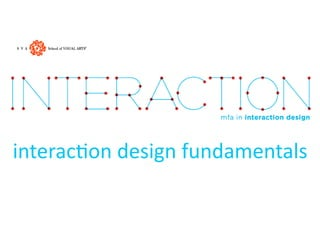Successfully reported this slideshow.

63

Share×
1 of 34
1 of 34

63

Share

#### Description

A 16-minute talk about sketching for interaction design, with personal and professional examples. Sound quality is a little rough.

#### Transcript

1. !quot;#\$%&'(quot;)*+,( -\$.,/0%&'(quot;)*+,( 1&,.2quot;/3&'
2. 402%5,*60.2(7quot;0.8 graphpaper.com
3. !quot;#\$%&'()quot;*+\$,!-quot;*./quot;+&0\$quot;#&1,
4. 5\$.,/0%&'
5. -\$.,/0(FG.5*#+3*&(-6./,2+AH >*@7%<.#%,8 9 :.5,+2.5 9 ;*2<5 9 ;0%,.)*quot;2< 9 =.&/%# 9 -0quot;26%. 9 4*##quot;'. 9 >*?@7%<.#%,8(-/0.Aquot;3/5(*2(;%2.B2quot;A. 9 C%@7%<.#%,8(-/0.Aquot;3/5(*2(;%2.B2quot;A. 9 D*<.#E(4*A6E(*2(D*/\$@+6 9 7+&/3*&quot;#(=2*,*,86. C%'0@7%<.#%,8
6. -\$.,/0(FG.5*#+3*&(-6./,2+AH >*@7%<.#%,8 9 :.5,+2.5 9 ;*2<5 9 ;0%,.)*quot;2< 9 =.&/%# 9 -0quot;26%. 9 4*##quot;'. 9 >*?@7%<.#%,8(-/0.Aquot;3/5(*2(;%2.B2quot;A. 9 C%@7%<.#%,8(-/0.Aquot;3/5(*2(;%2.B2quot;A. 9 D*<.#E(4*A6E(*2(D*/\$@+6 9 7+&/3*&quot;#(=2*,*,86. C%'0@7%<.#%,8
7. 5\$.,/0%&' (
8. 5\$.,/0%&' I(,0%&\$%&'
9. !0%&\$%&'( quot;)*+,(,0.( <.5%'&( 62*)#.A 1A6#.A.&3&'( ,0.(<.5%'&( 5*#+3*& J((((((((((((((((((KJ((((((((((((((((((LJ((((((((((((((((((MJ((((((((((((((((((NJ(((((((((((((((((((OJJ !%A.(5%&/.().'%&&%&'(*B(5.55%*&P
10. !0%&\$%&'( quot;)*+,(,0.( <.5%'&( 62*)#.A 1A6#.A.&3&'( ,0.(<.5%'&( 5*#+3*& J((((((((((((((((((KJ((((((((((((((((((LJ((((((((((((((((((MJ((((((((((((((((((NJ(((((((((((((((((((OJJ !%A.(5%&/.().'%&&%&'(*B(5.55%*&P
11. !0%&\$%&'( quot;)*+,(,0.( <.5%'&( 62*)#.A =2*)#.A -*#+3*& 1A6#.A.&3&'( ,0.(<.5%'&( 5*#+3*& J((((((((((((((((((KJ((((((((((((((((((LJ((((((((((((((((((MJ((((((((((((((((((NJ(((((((((((((((((((OJJ !%A.(5%&/.().'%&&%&'(*B(5.55%*&P
12. !0quot;&\$5Q 402%5,*60.2(7quot;0.8 graphpaper.com

#### Description

A 16-minute talk about sketching for interaction design, with personal and professional examples. Sound quality is a little rough.

#### Transcript

1. !quot;#\$%&'(quot;)*+,( -\$.,/0%&'(quot;)*+,( 1&,.2quot;/3&'
2. 402%5,*60.2(7quot;0.8 graphpaper.com
3. !quot;#\$%&'()quot;*+\$,!-quot;*./quot;+&0\$quot;#&1,
4. 5\$.,/0%&'
5. -\$.,/0(FG.5*#+3*&(-6./,2+AH >*@7%<.#%,8 9 :.5,+2.5 9 ;*2<5 9 ;0%,.)*quot;2< 9 =.&/%# 9 -0quot;26%. 9 4*##quot;'. 9 >*?@7%<.#%,8(-/0.Aquot;3/5(*2(;%2.B2quot;A. 9 C%@7%<.#%,8(-/0.Aquot;3/5(*2(;%2.B2quot;A. 9 D*<.#E(4*A6E(*2(D*/\$@+6 9 7+&/3*&quot;#(=2*,*,86. C%'0@7%<.#%,8
6. -\$.,/0(FG.5*#+3*&(-6./,2+AH >*@7%<.#%,8 9 :.5,+2.5 9 ;*2<5 9 ;0%,.)*quot;2< 9 =.&/%# 9 -0quot;26%. 9 4*##quot;'. 9 >*?@7%<.#%,8(-/0.Aquot;3/5(*2(;%2.B2quot;A. 9 C%@7%<.#%,8(-/0.Aquot;3/5(*2(;%2.B2quot;A. 9 D*<.#E(4*A6E(*2(D*/\$@+6 9 7+&/3*&quot;#(=2*,*,86. C%'0@7%<.#%,8
7. 5\$.,/0%&' (
8. 5\$.,/0%&' I(,0%&\$%&'
9. !0%&\$%&'( quot;)*+,(,0.( <.5%'&( 62*)#.A 1A6#.A.&3&'( ,0.(<.5%'&( 5*#+3*& J((((((((((((((((((KJ((((((((((((((((((LJ((((((((((((((((((MJ((((((((((((((((((NJ(((((((((((((((((((OJJ !%A.(5%&/.().'%&&%&'(*B(5.55%*&P
10. !0%&\$%&'( quot;)*+,(,0.( <.5%'&( 62*)#.A 1A6#.A.&3&'( ,0.(<.5%'&( 5*#+3*& J((((((((((((((((((KJ((((((((((((((((((LJ((((((((((((((((((MJ((((((((((((((((((NJ(((((((((((((((((((OJJ !%A.(5%&/.().'%&&%&'(*B(5.55%*&P
11. !0%&\$%&'( quot;)*+,(,0.( <.5%'&( 62*)#.A =2*)#.A -*#+3*& 1A6#.A.&3&'( ,0.(<.5%'&( 5*#+3*& J((((((((((((((((((KJ((((((((((((((((((LJ((((((((((((((((((MJ((((((((((((((((((NJ(((((((((((((((((((OJJ !%A.(5%&/.().'%&&%&'(*B(5.55%*&P
12. !0quot;&\$5Q 402%5,*60.2(7quot;0.8 graphpaper.com

## More Related Content

### Related Books

Free with a 30 day trial from Scribd

See all

### Related Audiobooks

Free with a 30 day trial from Scribd

See all